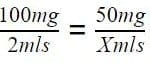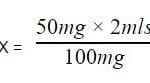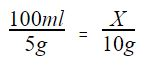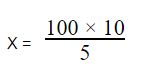21st November 2018
Many now include a calculation station as part of their Multiple Mini Interview (MMI). For example, Keele also has a separate maths test as part of their interview process. In this blog, we will show you how to undertake drug calculation questions and provide some examples.

### Why Are Drug Calculations Used?

The rationale for assessing calculation skills according to Keele is as follows:

“It is very important that doctors are able to use simple maths to solve problems during their work. Incorrect calculations can have very serious consequences for patient safety. Therefore we have introduced a maths test to ensure that students accepted to study medicine are able to undertake the type of calculation needed…”

“…the questions on the interview day will all be related to clinical scenarios, although no clinical knowledge is required to answer the questions..”

“…Simple calculators may be used and these will be supplied.”

“..TThe clinical maths test consists of 20 maths questions to be completed in 30 minutes. Those who qualify for the UCAT special educational needs [UCATSEN] test will be given extra time on the day. There are no trick questions and the answers are all realistic.”

Birmingham say this about their calculation station, which forms part of a wider data interpretation station:

“We expect you will have 10 minutes to complete one or two tasks, and all applicants will complete the task at the same time, via a virtual software platform off camera. You will be asked to undertake simple mathematical calculations involving data that has clinical relevance.”

Whether you are allowed to use a calculator or not depends on the Medical School.

This blog will take you through some of the more clinically useful calculations that could feature in these stations.

## Turn Medical School interviews into offers!

Explore all of our doctor-created interview prep in one place

### How Do You Convert Between Units?

In clinical practice one of the most useful type calculations to know how to perform is converting between units.

Example:

You prescribe a patient 1 g of paracetamol. How many milligrams is this?

A: 1 g = 1000 mg

### Drugs Calculations Summary Table

 Unit Gram Equivalents Exponential Form Kilogram (kg) 1000.0 g 103 g Gram (g) 1.0 g 1 g Milligram (mg) 0.001 g 10-3 g Microgram (μg) 0.000,001 g 10-6 g Nanogram (ng) 0.000,000,001 g 10-9 g

You may find it helpful to write out the number with a decimal point after it. The decimal point moves three places to the left for every unit you move up (e.g. from grams to kilograms and three places to the right every time you move down a unit e.g from grams to milligrams. You need to move the decimal point six places if you move through two units (e.g from milligrams to nanograms).

### How Do You Work Out Concentrations?

Some drug calculations may involve working out the volume of solution needed to give the required dose of a drug. One way to tackle these types of questions is to set up a simple equation where X is the variable you are trying to find. For example:

You are asked to give a patient weighing 50 kg a 1 mg/kg IV injection. The syringe contains 100 mg in 2 ml. What volume of the solution in the syringe do you need to give?

The patient weighs 50 kg and you need to give 1 mg per kg. 1 x 50 mg = 50 mg

Therefore you need to give a total dose of 50 mg.

The question states there is 100 mg in 2 ml. You need to give 50 mg and therefore need to find out what volume contains 50 mg.

You can set up an equation where X is the volume of the solution you are trying to find:Rearrange the equation to find XX= 1ml

### Percentage Concentrations

Some questions may give you a concentration in the form of a percentage, for example, a patient is given a 5% solution of a drug. By convention, this means there are 5 g of the drug in every 100 mL. You can then use this information to work out what volume of a solution you need to give in order to give the patient the correct dose of a drug.

An example of this type of question is given below:

You are asked to give a patient 10 g of the drug WondaDrug. This comes as a 5% solution in bags containing 1 L. What volume of the 1 L bag do you need to give?

A: 5% means there are 5 g of WondaDrug in every 100 mL.Rearrange the equation to find XX= 200 mL

Here is another example:

You are asked to give a patient 1ml of 1% lidocaine. How many mg are you giving the patient?

A: 1% means there is 1 g in 100 mL.

Therefore there is 1000 mg in 100 mL

Therefore there is 10 mg in every 1 mL

Resultantly you are administering 10mg of lidocaine to the patient.

Keele have also released a document with some practice questions with complete worked answers. These will give you an idea of the level of difficulty you can expect. You should practice these timed.

You can find these here.